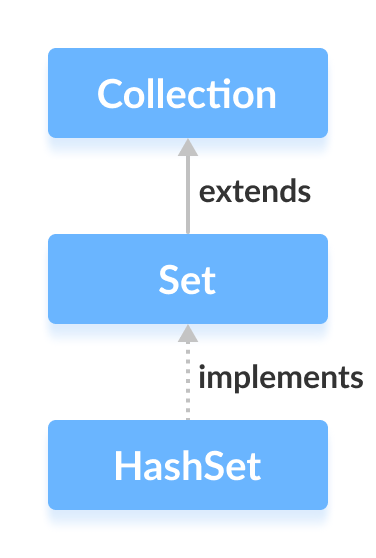# Java HashSet Class

In this tutorial, we will learn about the Java HashSet class. We will learn about different hash set methods and operations with the help of examples.

The `HashSet` class of the Java Collections framework provides the functionalities of the hash table data structure.

It implements the Set interface.## Creating a HashSet

In order to create a hash set, we must import the `java.util.HashSet` package first.

Once we import the package, here is how we can create hash sets in Java.

``````// HashSet with 8 capacity and 0.75 load factor
HashSet<Integer> numbers = new HashSet<>(8, 0.75);
``````

Here, we have created a hash set named `numbers`.

Notice, the part new `HashSet<>(8, 0.75)`. Here, the first parameter is capacity, and the second parameter is loadFactor.

• capacity - The capacity of this hash set is 8. Meaning, it can store 8 elements.
• loadFactor - The load factor of this hash set is 0.6. This means, whenever our hash set is filled by 60%, the elements are moved to a new hash table of double the size of the original hash table.

It's possible to create a hash table without defining its capacity and load factor. For example,

``````// HashSet with default capacity and load factor
HashSet<Integer> numbers1 = new HashSet<>();
``````

By default,

• the capacity of the hash set will be 16
• the load factor will be 0.75

## Methods of HashSet

The `HashSet` class provides various methods that allow us to perform various operations on the set.

## Insert Elements to HashSet

• `add()` - inserts the specified element to the set
• `addAll()` - inserts all the elements of the specified collection to the set

For example,

``````import java.util.HashSet;

class Main {
public static void main(String[] args) {
HashSet<Integer> evenNumber = new HashSet<>();

System.out.println("HashSet: " + evenNumber);

HashSet<Integer> numbers = new HashSet<>();

System.out.println("New HashSet: " + numbers);
}
}
``````

Output

```HashSet: [2, 4, 6]
New HashSet: [2, 4, 5, 6]
```

## Access HashSet Elements

To access the elements of a hash set, we can use the `iterator()` method. In order to use this method, we must import the `java.util.Iterator` package. For example,

``````import java.util.HashSet;
import java.util.Iterator;

class Main {
public static void main(String[] args) {
HashSet<Integer> numbers = new HashSet<>();
System.out.println("HashSet: " + numbers);

// Calling iterator() method
Iterator<Integer> iterate = numbers.iterator();
System.out.print("HashSet using Iterator: ");
// Accessing elements
while(iterate.hasNext()) {
System.out.print(iterate.next());
System.out.print(", ");
}
}
}
``````

Output

```HashSet: [2, 5, 6]
HashSet using Iterator: 2, 5, 6,
```

## Remove Elements

• `remove()` - removes the specified element from the set
• `removeAll()` - removes all the elements from the set

For example,

``````import java.util.HashSet;

class Main {
public static void main(String[] args) {
HashSet<Integer> numbers = new HashSet<>();
System.out.println("HashSet: " + numbers);

// Using remove() method
boolean value1 = numbers.remove(5);
System.out.println("Is 5 removed? " + value1);

boolean value2 = numbers.removeAll(numbers);
System.out.println("Are all elements removed? " + value2);
}
}
``````

Output

```HashSet: [2, 5, 6]
Is 5 removed? true
Are all elements removed? true
```

## Set Operations

The various methods of the `HashSet` class can also be used to perform various set operations.

## Union of Sets

To perform the union between two sets, we can use the `addAll()` method. For example,

``````import java.util.HashSet;

class Main {
public static void main(String[] args) {
HashSet<Integer> evenNumbers = new HashSet<>();
System.out.println("HashSet1: " + evenNumbers);

HashSet<Integer> numbers = new HashSet<>();
System.out.println("HashSet2: " + numbers);

// Union of two set
System.out.println("Union is: " + numbers);
}
}

``````

Output

```HashSet1: [2, 4]
HashSet2: [1, 3]
Union is: [1, 2, 3, 4]
```

## Intersection of Sets

To perform the intersection between two sets, we can use the `retainAll()` method. For example

``````import java.util.HashSet;

class Main {
public static void main(String[] args) {

HashSet<Integer> evenNumbers = new HashSet<>();
System.out.println("HashSet2: " + evenNumbers);

// Intersection of two sets
System.out.println("Intersection is: " + evenNumbers);
}
}
``````

Output

```HashSet1: [2, 3]
HashSet2: [2, 4]
Intersection is: 
```

## Difference of Sets

To calculate the difference between the two sets, we can use the `removeAll()` method. For example,

``````import java.util.HashSet;

class Main {
public static void main(String[] args) {

HashSet<Integer> oddNumbers = new HashSet<>();
System.out.println("HashSet2: " + oddNumbers);

// Difference between HashSet1 and HashSet2
}
}
``````

Output

```HashSet1: [2, 3, 5]
HashSet2: [1, 3, 5]
Difference: 
```

## Subset

To check if a set is a subset of another set or not, we can use the `containsAll()` method. For example,

``````import java.util.HashSet;

class Main {
public static void main(String[] args) {
HashSet<Integer> numbers = new HashSet<>();
System.out.println("HashSet1: " + numbers);

// Check if primeNumbers is a subset of numbers
System.out.println("Is HashSet2 is subset of HashSet1? " + result);
}
}
``````

Output

```HashSet1: [1, 2, 3, 4]
HashSet2: [2, 3]
Is HashSet2 is a subset of HashSet1? true
```

## Other Methods Of HashSet

Method Description
`clone()` Creates a copy of the `HashSet`
`contains()` Searches the `HashSet` for the specified element and returns a boolean result
`isEmpty()` Checks if the `HashSet` is empty
`size()` Returns the size of the `HashSet`
`clear()` Removes all the elements from the `HashSet`

## Why HashSet?

In Java, `HashSet` is commonly used if we have to access elements randomly. It is because elements in a hash table are accessed using hash codes.

The hashcode of an element is a unique identity that helps to identify the element in a hash table.

`HashSet` cannot contain duplicate elements. Hence, each hash set element has a unique hashcode.

Note: HashSet is not synchronized. That is if multiple threads access the hash set at the same time and one of the threads modifies the hash set. Then it must be externally synchronized.